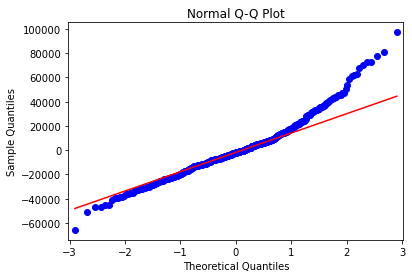# Normality in Error Term: Q-Q Plot and Jarque-Bera Test in Python

Last Update: February 21, 2022

Normality in Error Term: Q-Q Plot in Python can be done using `statsmodels` package `qqplot` function found within `statsmodels.api` module and `matplotlib` package `plot` function found within `matplotlib.pyplot` module for evaluating whether points comparing linear regression residuals sample quantiles and normal distribution theoretical quantiles are within quantiles regression line fit. Main parameters within `qqplot` function are `data` with model residuals, `dist` with comparison probability distribution and `line` with quantiles regression line fit, regression line fit, standardized line or 45-degree line options.

Normality in Error Term: Jarque-Bera Test in Python can be done using `statsmodels` package `jarque_bera` function found within `statsmodels.stats.api` module for evaluating whether linear regression residuals skewness and excess kurtosis are equal to zero. Main parameter within `jarque_bera` function is `resid` with model residuals.

As example, we can do residuals Q-Q plot and Jarque-Bera test from multiple linear regression of house price explained by its lot size and number of bedrooms using data included within `AER` R package `HousePrices` object .

First, we import `statsmodels` package for data downloading, multiple linear regression fitting, Q-Q plot and Jarque-Bera test, `scipy` package for normal probability distribution and `matplotlib` for Q-Q plot .

``````In :
import statsmodels.api as sm
import statsmodels.formula.api as smf
import statsmodels.stats.api as sms
import scipy.stats as st
import matplotlib.pyplot as plt
``````

Second, we create `houseprices` data object using `get_rdataset` function and display first five rows and three columns of data using `print` function and `head` data frame method to view its structure.

``````In :
houseprices = sm.datasets.get_rdataset(dataname="HousePrices", package="AER", cache=True).data
``````
``````
Out :
price  lotsize  bedrooms
0  42000.0     5850         3
1  38500.0     4000         2
2  49500.0     3060         3
3  60500.0     6650         3
4  61000.0     6360         2

``````

Third, we fit multiple linear regression with `ols` function using variables within `houseprices` data object and store results within `mlr` object. Within `ols` function, parameter `formula="price ~ lotsize + bedrooms"` fits model where house price is explained by its lot size and number of bedrooms.

``````In :
mlr = smf.ols(formula="price ~ lotsize + bedrooms", data=houseprices).fit()
``````

Fourth, we get residuals from `mlr` multiple linear regression results object and store them within `res` object.

``````In :
res = mlr.resid
``````

Fifth, we do normal Q-Q plot using `qqplot` and `plot` functions. Within `qqplot` function, parameters `data=res` includes model residuals, `dist=st.norm` includes `scipy` package normal probability distribution for comparison and `line="q"` includes quantiles regression line fit.

``````In :
fig = sm.qqplot(data=res, dist=st.norm, line="q")
plt.title("Normal Q-Q Plot")
plt.show()
``````
``Out :``Figure 1. Residuals normal Q-Q plot from multiple linear regression of house price explained by its lot size and number of bedrooms.

Sixth, we do Jarque-Bera test using `jarque_bera` function, store results within `jbtest` object and print its `JB` test statistic and `JBpv` test statistic p-value results. Within `jarque_bera` function, parameter `resids = res` includes model residuals.

``````In :
jbtest = sms.jarque_bera(resids = res)
print("JB:", jbtest, "JBpv:", jbtest)
``````
``````Out :
JB: 146.85443903231146 JBpv: 1.2911114798088417e-32
``````

Courses

My online courses are hosted at Teachable website.

For more details on this concept, you can view my Linear Regression in Python Course.

 Data Description: Sales prices of houses sold in the city of Windsor, Canada, during July, August and September, 1987.

Original Source: Anglin, P., and Gencay, R. (1996). Semiparametric Estimation of a Hedonic Price Function. Journal of Applied Econometrics, 11, 633–648.

 statsmodels Python package: Seabold, Skipper, and Josef Perktold. (2010). “statsmodels: Econometric and statistical modeling with python”. Proceedings of the 9th Python in Science Conference.

scipy Python package: Pauli Virtanen, Ralf Gommers, Travis E. Oliphant, Matt Haberland, Tyler Reddy, David Cournapeau, Evgeni Burovski, Pearu Peterson, Warren Weckesser, Jonathan Bright, Stéfan J. van der Walt, Matthew Brett, Joshua Wilson, K. Jarrod Millman, Nikolay Mayorov, Andrew R. J. Nelson, Eric Jones, Robert Kern, Eric Larson, CJ Carey, İlhan Polat, Yu Feng, Eric W. Moore, Jake VanderPlas, Denis Laxalde, Josef Perktold, Robert Cimrman, Ian Henriksen, E.A. Quintero, Charles R Harris, Anne M. Archibald, Antônio H. Ribeiro, Fabian Pedregosa, Paul van Mulbregt, and SciPy 1.0 Contributors. (2020). SciPy 1.0: Fundamental Algorithms for Scientific Computing in Python. Nature Methods, 17(3), 261-272.

matplotlib Python package: John D. Hunter. (2007). Matplotlib: A 2D Graphics Environment. Computing in Science & Engineering, 9, 90-95.

+